# 2 Theoretical Model

We consider a 1-D collisionless, four-component plasma consisting of cool inertial background electrons (at temperature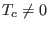), mobile cool positrons (or electron holes; at temperature), inertialess hot suprathermal electrons modeled by a-distribution (at temperature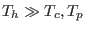), and uniformly distributed stationary ions.

The cool electrons and positrons are governed by the following fluid equations: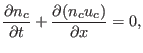(1)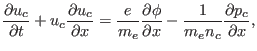(2)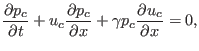(3)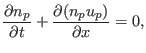(4)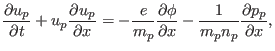(5)(6)

where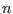,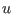and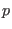are the number density, the velocity and the pressure of the cool electrons and positrons (denoted by indices c' and p', respectively),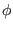is the electrostatic wave potential,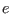the elementary charge,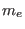the electron mass,the positron mass, and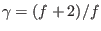denotes the specific heat ratio for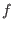degrees of freedom. For the adiabatic cool electrons and positrons in one-dimensional (), we get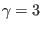. Through this paper, we assume that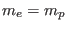.

Following Eq. 1 in Ref. , the-distribution expression is obtained for the number density of the hot suprathermal electrons:(7)

where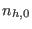and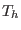are the equilibrium number density and the temperature of the hot electrons, respectively,the Boltzmann constant, and the spectral indexmeasures the deviation from thermal equilibrium. For reality of the characteristic modified thermal velocity,, the spectral index must take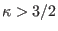. The suprathermality is measured by the spectral index, describing how it deviates from a Maxwellian distribution, i.e., low values ofare associated with a significant suprathermality; on the other hand, a Maxwellian distribution is recovered in the limit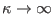.

The ions are assumed to be immobile in a uniform state, i.e.,const. at all times, where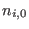is the undisturbed ion density. The plasma is quasi-neutral at equilibrium, so, that implies(8)

where we have defined the hot-to-cool electron density ratio as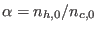, and the positron-to-cool electron density ratio as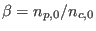, while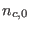and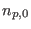are the equilibrium number densities of the cool electrons and positrons, respectively. Electrostatic waves are weakly damped in the range of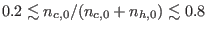[26,27,28,29], so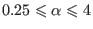. This region may permit the propagation of nonlinear electrostatic structures. As the positron fraction has been measured to be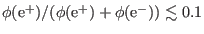in low energy solar wind observations [1,2,3,5] and-0.1 in some laser-plasma experiences [14,15], we assume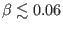.

All four components are coupled via the Poisson's equation: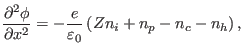(9)

where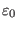is the permittivity constant.

Scaling by appropriate quantities, we arrive at a fluid system of our model in a dimensionless form for the cool electrons and the positrons, respectively: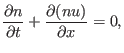(10)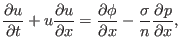(11)(12)(13)(14)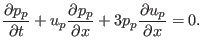(15)

The dimensionless Poisson's equation takes the following form:(16)

whereanddenote the fluid density variables of the cool electrons and positrons normalized with respect toand, respectively,andthe velocity variables of the cool electrons and positrons scaled by the hot electron thermal speed,and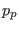the pressure variables of the cool electrons and positrons normalized with respect toand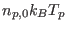, respectively, and the wave potentialby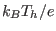, time and space scaled by the plasma periodand the characteristic length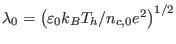, respectively. We have defined the cool-to-hot electron temperature ratio as, and the positron-to-hot electron temperature ratio as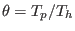. Landau damping is minimized if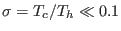[26,27,28], and the same for the cool positrons, i.e.,(see Ref. ), and typically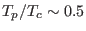in some laser-plasma experiences .

Ashkbiz Danehkar
2018-03-28